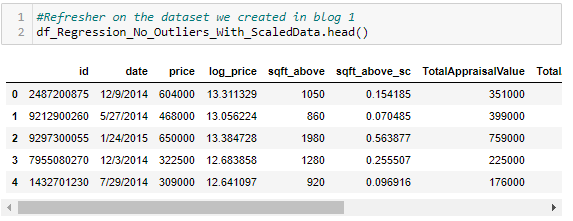# IntroductionKing County Data + Scraped Data From King County Tax Assessors

# 1.Creating Our Model

`# Import appropriate Statsmodel Librariesimport statsmodels.api as sm# Separate Our Variables# Get our Target Variable – Actual Price Reacheddf_Regresion_Target = df_Regression_No_Outliers_With_ScaledData['log_price']#Get our Features - Remove any columns not used to predict our target.df_Regresion_Features = df_Regression_No_Outliers_With_ScaledData.drop(columns=['id', 'date', 'price','log_price',‘sqft_above',  'TotalAppraisalValue'])# Use Features to create y interceptpredictors_int = sm.add_constant(df_Regresion_Features)# Feed Features and intercept to Model Method for Model creationmodel = sm.OLS(df_Regresion_Target,predictors_int).fit()#View modelmodel.summary()`
`#Run Predictions using the modeldf_predictedPrice = model.predict(predictors_int)#Create Dataframe From Predictionsf_predictions = pd.DataFrame(df_predictedPrice, columns=["log_price_Predicted"])df_predictions["price_Predicted"] = np.exp(df_predictions["log_price_Predicted"])df_predictions["log_Residuals"] = model.resid#Combine Predictions with Original datafromedf_predictions = df_predictions.reset_index(drop=True)df_Regression_No_Outliers_With_ScaledData = df_Regression_No_Outliers_With_ScaledData.reset_index(drop=True)df_Regression_No_Outliers_With_ScaledData = pd.concat([df_Regression_No_Outliers_With_ScaledData, df_predictions], axis=1)df_Regression_No_Outliers_With_ScaledData['price'] = df_Regression_No_Outliers_With_ScaledData['price'].astype(float)df_Regression_No_Outliers_With_ScaledData["price_Residuals"] =  df_Regression_No_Outliers_With_ScaledData.apply(lambda x: x['price_Predicted'] - x['price'], axis=1)#Reorder columns, put prices next to each otherdf_Regression_No_Outliers_With_ScaledData = df_Regression_No_Outliers_With_ScaledData[['id', 'date', 'price', 'price_Predicted', 'price_Residuals', 'log_price', 'log_price_Predicted','log_Residuals','sqft_above', 'Sch_d_Top15','Sch_d_Top30', 'Sch_d_Top60', 'TotalAppraisalValue', 'sqft_above_sc','TotalAppraisalValue_sc',]]#Display Resultsdf_Regression_No_Outliers_With_ScaledData.head()#Run Predictions using the modeldf_predictedPrice = model.predict(predictors_int)#Create Dataframe From Predictionsdf_predictions = pd.DataFrame(df_predictedPrice, columns=["log_price_Predicted"])df_predictions["price_Predicted"] = df_predictions.apply(lambda x: np.exp(x['log_price_Predicted']), axis=1)df_predictions["log_Residuals"] = model.resid#Combine Predictions with Original datafrome, create Residuals column by substracting Actual From Predicteddf_predictions = df_predictions.reset_index(drop=True)df_Regression_No_Outliers_With_ScaledData = df_Regression_No_Outliers_With_ScaledData.reset_index(drop=True)df_Regression_No_Outliers_With_ScaledData = pd.concat([df_Regression_No_Outliers_With_ScaledData, df_predictions], axis=1)df_Regression_No_Outliers_With_ScaledData['price'] = df_Regression_No_Outliers_With_ScaledData['price'].astype(float)df_Regression_No_Outliers_With_ScaledData["price_Residuals"] =  df_Regression_No_Outliers_With_ScaledData.apply(lambda x: x['price_Predicted'] - x['price'], axis=1)df_Regression_No_Outliers_With_ScaledData["price_Residuals_abs"] =  abs(df_Regression_No_Outliers_With_ScaledData["price_Residuals"])#Reorder columns, put prices next to each otherdf_Regression_No_Outliers_With_ScaledData = df_Regression_No_Outliers_With_ScaledData[['id', 'date', 'price', 'price_Predicted', 'price_Residuals','price_Residuals_abs','log_price','log_price_Predicted','log_Residuals','sqft_above', 'Sch_d_Top15','Sch_d_Top30', 'Sch_d_Top60', 'TotalAppraisalValue','sqft_above_sc','TotalAppraisalValue_sc',]]#Display Resultsdf_Regression_No_Outliers_With_ScaledData.head()`
`#Graph our residuals against our predictions, this will give us a sense if our model is off for certain priced homesplt.scatter(df_predictions["price_Predicted"], df_Regression_No_Outliers_With_ScaledData["price"])plt.xlabel("Predicted Price")]plt.xticks(ticks=(200000, 400000, 700000, 900000),labels= ('\$200k', '\$400k', '\$700k', '\$900k'))plt.ylabel("Residual")plt.yticks(ticks=(200000, 400000, 700000, 900000),labels= ('\$200k', '\$400k', '\$700k', '\$900k'))plt.plot([200000, 900000], [200000, 900000], color = 'red', linewidth = 2)plt.show()`
`#Graph our residuals against our predictions, this will give us a sense if our modle is off for certain priced homesplt.scatter(df_predictions["price_Predicted"], df_Regression_No_Outliers_With_ScaledData["price_Residuals"])# plt.plot(Model1.predict(df_X_train), [0 for i in range(len(df_X_train))]);plt.xlabel("Predicted Price")plt.xticks(ticks=(300000, 500000, 700000, 900000, 1200000),labels= ('\$300k', '\$500k', '\$700k', '\$900k','\$1.2M'))plt.ylabel("Residual")plt.yticks(ticks=(-300000, -100000, 0, 300000, 500000),labels= ('\$-300k', '\$-100k', '\$0k', '\$300k','\$500k'))plt.show()`

# 2. Using Your Model Again

`# Create the average home used the average from each featured = {'a_price'[df_Regression_No_Outliers_With_ScaledData['price'].mean()]}df_The_Average_Home = pd.DataFrame(data=d)df_The_Average_Home['a_log_price'] = df_Regression_No_Outliers_With_ScaledData['log_price'].mean()df_The_Average_Home['a_sqft_above'] = df_Regression_No_Outliers_With_ScaledData['sqft_above'].mean()df_The_Average_Home['a_Sch_d_Top15'] = 0df_The_Average_Home['a_Sch_d_Top30'] = 0df_The_Average_Home['a_Sch_d_Top60'] = 1df_The_Average_Home['a_TotalAppraisalValue'] = df_Regression_No_Outliers_With_ScaledData['TotalAppraisalValue'].mean()df_The_Average_Home['a_sqft_above_sc'] = df_Regression_No_Outliers_With_ScaledData['sqft_above_sc'].mean()df_The_Average_Home['a_TotalAppraisalValue_sc'] = df_Regression_No_Outliers_With_ScaledData['TotalAppraisalValue_sc'].mean()# Display the average homedf_The_Average_Home.head()`
`# Create dataframe to feed into scaler, make sure not to feed scaler your categorical datadf_New_Data_To_Be_Scaled = df_The_Average_Home[["a_sqft_above","a_TotalAppraisalValue"]]# Feed data into scales using the .transform() method vs. .fit_transform()Newly_scaled_Data = scaler.transform(df_New_Data_To_Be_Scaled)# Create dataframe from newly scaled valuesdf_Newly_scaled_Data = pd.DataFrame(Newly_scaled_Data, columns=["sqft_above_sc","TotalAppraisalValue_sc"])df_Newly_scaled_Data = df_Newly_scaled_Data.reset_index(drop=True)# Get caegorical values to feed into new predictiondf_Average_Categoricals = df_The_Average_Home[['a_Sch_d_Top15', 'a_Sch_d_Top30','a_Sch_d_Top60']]#Bring two sets of data together prior to feeding dataframedf_Average_Categoricals = df_Average_Categoricals.reset_index(drop=True)df_New_Row_For_Prediction = pd.concat([df_Newly_scaled_Data, df_Average_Categoricals], axis=1)#add contanct in position 1df_New_Row_For_Prediction.insert (0, "constant", 1)# Use Features to create interceptnew_predictors_int = sm.add_constant(df_New_Row_For_Prediction)Newpredicted_value_log = model.predict(df_New_Row_For_Prediction)Newpredicted_value = np.exp(Newvalue_log)Newpredicted_value`

--

--